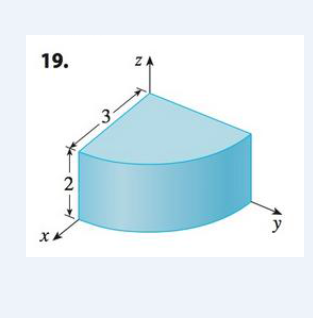Chapter 15.8, Problem 19E

Chapter
Section
Textbook Problem

Set up the triple integral of an arbitrary of an arbitrary continuous function f(x, y, z) in cylindrical or spherical coordinates over the solid shown.To determine

To setup: The triple integral in cylindrical or spherical coordinates.

Explanation

Given:

In the graph, x and z coordinate values are given. Thus, it is more appropriate and easier to use the cylindrical coordinates to set up the triple integral.

Formula used:

If f is a cylindrical region E given by h1(θ)rh2(θ),αθβ, u1(rcosθ,rsinθ)zu1(rcosθ,rsinθ) where 0βα2π , then,

Ef(x,y,z)dV=αβh1(θ)h2(θ)u1(rcosθ,rsinθ)u2(rcosθ,rsinθ<

Still sussing out bartleby?

Check out a sample textbook solution.

See a sample solution

The Solution to Your Study Problems

Bartleby provides explanations to thousands of textbook problems written by our experts, many with advanced degrees!

Get Started

Find more solutions based on key concepts